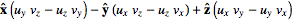# Simple Cross Product Equation Question

Gold Member

## Homework Statement

This is a general question about the equation.
So, I know that the cross product requires a vector in at least 3 dimensions crossed with another.

Here is the formula that I am using:
uxv =My problem is the negative/positive sign orientation in front of the y element and z element.
I've seen equations for this where there exists only addition between the x,y, and z elements in a cross product. And my teacher tried to explain it to me, and I softly understood, but I want to make sure I know what's going on.

Why does the cross product equation sometimes have all positive signs between elements, but the one that I use (and works) has a negative and then a positive sign?

SteamKing
Staff Emeritus
Homework Helper

## Homework Statement

This is a general question about the equation.
So, I know that the cross product requires a vector in at least 3 dimensions crossed with another.

Here is the formula that I am using:
uxv =My problem is the negative/positive sign orientation in front of the y element and z element.
I've seen equations for this where there exists only addition between the x,y, and z elements in a cross product. And my teacher tried to explain it to me, and I softly understood, but I want to make sure I know what's going on.

Why does the cross product equation sometimes have all positive signs between elements, but the one that I use (and works) has a negative and then a positive sign?

It's not clear from what source you are deriving these different formulas for the cross product.

Here is an article with various formulas for computing it:

http://en.wikipedia.org/wiki/Cross_product

I tend to favor the formula which uses the expansion of the 3 x 3 formal determinant. It is easy to remember and expand, and the negative signs take care of themselves.

•RJLiberator
Gold Member
Ah, wiki. Why did I not think of that? :p.

This appears to have my answer. I will post back if I get confused further.

Thank you.

SteamKing
Staff Emeritus
Homework Helper
Ah, wiki. Why did I not think of that? :p.

This appears to have my answer. I will post back if I get confused further.

Thank you.

Wiki has some surprisingly good math articles, particularly on the practical aspects of calculation. You can always check what's there with Wolfram's MathWorld site:

http://mathworld.wolfram.com/

•RJLiberator

there is a better video that uses matrix(kin of like craters rule) somewhere. I'll find it later

•RJLiberator
SteamKing
Staff Emeritus
Homework Helper
there is a better video that uses matrix(kin of like craters rule) somewhere. I'll find it later

It's Cramer's Rule, not craters rule. Cramer's Rule is used to solve a system of linear equations using determinants.

The method used to expand the determinant of a 3 x 3 matrix is actually called the Rule of Sarrus. See

http://en.wikipedia.org/wiki/Rule_of_Sarrus

I am typing on a tablet.

Ray Vickson
Homework Helper
Dearly Missed

## Homework Statement

This is a general question about the equation.
So, I know that the cross product requires a vector in at least 3 dimensions crossed with another.

Here is the formula that I am using:
uxv =My problem is the negative/positive sign orientation in front of the y element and z element.
I've seen equations for this where there exists only addition between the x,y, and z elements in a cross product. And my teacher tried to explain it to me, and I softly understood, but I want to make sure I know what's going on.

Why does the cross product equation sometimes have all positive signs between elements, but the one that I use (and works) has a negative and then a positive sign?

## The Attempt at a Solution

Think of the three cyclic permuations XYZ, YZX, ZXY, obtained by taking the letter in the first position and looping it around to be last. So, starting from XYZ we take the X and move it around to last place, shoving the other two to the left---giving YZX. Similarly, we then take the Y and loop it around to be last, to get ZXY. Those orders give you the first parts of each of the three terms:
$$\vec{u} \times \vec{v} = \underbrace{\vec{e}_x ( u_y v_z \cdots)}_{XYZ} + \underbrace{\vec{e}_y(u_z v_x \cdots)}_{YZX} + \underbrace{\vec{e}_z (u_x v_y \cdots)}_{ZXY}$$
Now just complete each bracket ##( )## by putting in the interchange of the first term, with a - sign:
$$\vec{u} \times \vec{v} = \vec{e}_x (u_y v_z - u_z v_y) + \vec{e}_y ( u_z v_x - u_x v_z) + \vec{e}_z (u_x v_y - u_y v_x)$$
Now there are ##+## signs in front of each of the terms.

•RJLiberator